# MCQ Set Theory

Collection of Multiple choice questions and Answers (MCQs) focuses on “Set Theory” along with their solution and justification.

Let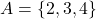and, then which of the following statements is correct?
(A)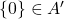in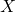(B)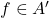with respect to(C)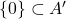with respect to(D)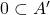with respect to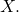We have,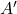in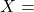The set of elements inwhich are not in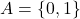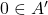inis false, because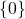is not an element ofin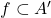inis false, because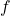is not an element ofininis correct, because the only element ofnamely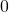also belongs toin.inis false, becauseis not a set.

If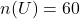,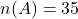,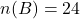andthen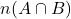is
(A) 9
(B) 8
(C) 6
(D) None of these

Explanation:
We have,Now,Two finite sets have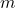and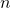elements, then total number of subsets of the first set is 56 more that the total number of subsets of the second. The values ofandare,
(A) 7, 6
(B) 6, 3
(D) 5, 1
(D) 8, 7

Solution: (B)
Since the two finite sets haveandelements, so number of subsets of these sets will be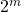and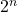respectively. According to the question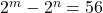putting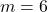,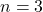, we get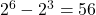or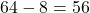If A has 3 elements and B has 6 elements, then the minimum number of elements in the set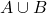is
(A) 6
(B) 3
(C)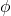(D) None of these

Clearly the number of elements inwill be minimum when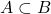. Hence the minimum number of elements inis the same as the number of elements in B, that is, 6.
Clearly the number of elements inwill be minimum when. Hence the minimum number of elements inis the same as the number of elements in